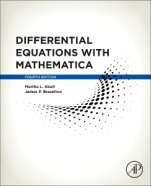### Logowanie

Zapomniałeś hasła?
Nowy klient

Jeżeli nie znalazłeś poszukiwanej książki, skontaktuj się z nami wypełniając formularz kontaktowy.

Ta strona używa plików cookies, by ułatwić korzystanie z serwisu. Mogą Państwo określić warunki przechowywania lub dostępu do plików cookies w swojej przeglądarce zgodnie z polityką prywatności.

### Informacje szczegółowe o książce# Differential Equations with Mathematica

##### ISBN 9780128047767

Autor: Abell, Martha L.Braselton, James P.

Wydawca: Elsevier

Dostępność: Wysyłka w ciągu 2-3 dni

Cena: 614,25 zł

 ISBN13: 9780128047767 Autor: Abell, Martha L.Braselton, James P. Oprawa: Paperback Rok Wydania: 2016-09-29 Tematy: PBKJ

Differential Equations with Mathematica, Fourth Edition is a supplementing reference which uses the fundamental concepts of the popular platform to solve (analytically, numerically, and/or graphically) differential equations of interest to students, instructors, and scientists.

Mathematica’s diversity makes it particularly well suited to performing calculations encountered when solving many ordinary and partial differential equations. In some cases, Mathematica’s built-in functions can immediately solve a differential equation by providing an explicit, implicit, or numerical solution. In other cases, mathematica can be used to perform the calculations encountered when solving a differential equation.

Because one goal of elementary differential equations courses is to introduce students to basic methods and algorithms so that they gain proficiency in them, nearly every topic covered this book introduces basic commands, also including typical examples of their application. A study of differential equations relies on concepts from calculus and linear algebra, so this text also includes discussions of relevant commands useful in those areas. In many cases, seeing a solution graphically is most meaningful, so the book relies heavily on Mathematica’s outstanding graphics capabilities.

Key Features Demonstrates how to take advantage of the advanced features of Mathematica 10 Introduces the fundamental theory of ordinary and partial differential equations using Mathematica to solve typical problems of interest to students, instructors, scientists, and practitioners in many fields Showcases practical applications and case studies drawn from biology, physics, and engineering Readership

All users of Mathematica who encounter differential equations in their research, in particular beginning users such as students, instructors, engineers, and professionals using Mathematica to solve and visualize solutions to differential equations

About the Author Martha L. Abell

Martha L. Abell and James P. Braselton are graduates of the Georgia Institute of Technology and the Ohio State University, respectively, and teach at Georgia Southern University, Statesboro where they have extensive experience in Mathematica-assisted instruction at both the undergraduate and graduate levels. In addition, they have given numerous presentations on Mathematica, throughout the United States and abroad. Other books by the authors include Differential Equations with Mathematica, Second Edition and Statistics with Mathematica.

Affiliations and Expertise

Georgia Southern University, Statesboro, USA

James P. Braselton

Martha L. Abell and James P. Braselton are graduates of the Georgia Institute of Technology and the Ohio State University, respectively, and teach at Georgia Southern University, Statesboro where they have extensive experience in Mathematica-assisted instruction at both the undergraduate and graduate levels. In addition, they have given numerous presentations on Mathematica, throughout the United States and abroad. Other books by the authors include Differential Equations with Mathematica, Second Edition and Statistics with Mathematica.

Affiliations and Expertise

Georgia Southern University, Statesboro, USA

Preface Introduction to Differential Equations First-Order Ordinary Differential Equations Applications of First-Order Ordinary Differential Equations Higher-Order Differential Equations Applications of Higher-Order Differential Equations Systems of Ordinary Differential Equations Applications of Systems of Ordinary Differential Equations Laplace Transform Methods Eigenvalue Problems and Fourier Series Partial Differential Equations Appendix: Getting Started The Mathematica Menu Bibliography Index

### Koszyk

Książek w koszyku: 0 szt.

Wartość zakupów: 0,00 zł

### Kontakt

Gambit
Centrum Oprogramowania
i Szkoleń Sp. z o.o.

Al. Pokoju 29b/22-24

31-564 Kraków

+48 12 414 3791

+48 12 414 3387

Siedziba Księgarni

ul. Kordylewskiego 1

31-542 Kraków

+48 12 410 5989

+48 12 414 3767

Wyślij e-mail

### Subskrypcje

Administratorem danych osobowych jest firma Gambit COiS Sp. z o.o. Na podany adres będzie wysyłany wyłącznie biuletyn informacyjny.

### Autoryzacja płatności